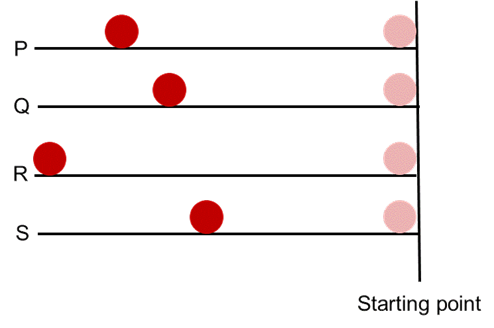# Contact and Non-Contact Forces

## •    Force is defined as an agent which changes or tends to change the state of rest or uniform motion of a body or changes its direction or shape. The SI unit of force is newton (N). In cgs system, force is measured in dyne. •    Force is the cause of motion of a stationary body, stopping, decreasing or increasing the speed of a body and change in the shape or size of a body. Force is further dived as Contact force: Contact force is defined as the force between two bodies when they are in physical contact. Contact forces can be frictional force, normal reaction, tension in strings and the force acting between two bodies when they collide.  Non-contact force: Non-contact force is defined as the force between two bodies when they are not in physical contact. Non-contact forces can be gravitational force, electrostatic force and magnetic force. •    On the basis of magnitude and direction of force, it is further classified as Balanced forces: Balanced forces are the forces which are equal in magnitude and opposite in direction. A stationary body under balanced forces remains stationary while a moving body keeps on moving with same velocity.Unbalanced forces: Unbalanced forces are unequal in magnitude and opposite in direction. A stationary body under unbalanced forces starts to move whereas the velocity of moving body may increase or decrease. The direction of motion of the moving body may also be changed.

To Access the full content, Please Purchase

• Q1

What will happen if the external unbalanced force on a moving car is removed?

Marks:1
##### Answer:

It will move with the uniform velocity it has acquired till then

##### Explanation:

A body moves due to unbalanced forces acting on it. If unbalanced force keep acting on a body, it will bring change in either in the direction or speed or shape of the body. If no unbalanced force acts on a body then body will continue its state of rest or motion with uniform velocity.

View Answer
• Q2

When a ball is thrown at a wall it rebounds. The effect of force is

Marks:1
##### Answer:

to change the direction of motion

##### Explanation:

When a ball is thrown on the wall or on the ground, it rebounds due to the reaction force of the surface on it. Thus, it changes the direction of motion of the ball.

View Answer
• Q3

How many minimum forces are needed to keep a body at equilibrium?

Marks:1

Two

##### Explanation:

A minimum of two balanced forces are required to keep a body at equilibrium.

View Answer
• Q4

Four balls are made to roll on four different surfaces. Their starting points are same but they stopped at different positions.If all the balls are rolled with the same force, the surface which has maximum friction is

Marks:1

S

##### Explanation:

Frictional force opposes motion. As large is the frictional force, quickly the moving object will come to rest, if external force supporting motion is removed.

View Answer
• Q5

Observe the given diagrams carefully and choose the option inferring the correct information.Marks:1

A is magnet

##### Explanation:

Repulsion occurs only between like poles of two magnets whereas attraction can occur between two unlike poles of magnet and a magnet and magnetic material, so repulsion is the surest test of magnetism.

View Answer